CAT  >  Test Level 3: Percentages

# Test Level 3: Percentages

Test Description

## 10 Questions MCQ Test Level-wise Tests for CAT | Test Level 3: Percentages

Test Level 3: Percentages for CAT 2023 is part of Level-wise Tests for CAT preparation. The Test Level 3: Percentages questions and answers have been prepared according to the CAT exam syllabus.The Test Level 3: Percentages MCQs are made for CAT 2023 Exam. Find important definitions, questions, notes, meanings, examples, exercises, MCQs and online tests for Test Level 3: Percentages below.
Solutions of Test Level 3: Percentages questions in English are available as part of our Level-wise Tests for CAT for CAT & Test Level 3: Percentages solutions in Hindi for Level-wise Tests for CAT course. Download more important topics, notes, lectures and mock test series for CAT Exam by signing up for free. Attempt Test Level 3: Percentages | 10 questions in 20 minutes | Mock test for CAT preparation | Free important questions MCQ to study Level-wise Tests for CAT for CAT Exam | Download free PDF with solutions
 1 Crore+ students have signed up on EduRev. Have you?
Test Level 3: Percentages - Question 1

### If a quantity A is successively increased by 20% for two times and another quantity B is successively increased by 10% for three times, then quantity A becomes 80% of quantity B. What percentage is the original value of quantity A as compared to the original value of quantity B?

Detailed Solution for Test Level 3: Percentages - Question 1

Option (4) is correct.
Let quantity A be x.
Two successive increase of 20% on x gives us 1.44x.
Let quantity B be y.
Three successive increase of 10% on y gives 1.331y.
Now, 80% of 1.331y = 1.44x
x = 0.739y
That is, x is 74% of y.

Test Level 3: Percentages - Question 2

### In an election, 3/5th of the voters promised to vote for X and the rest promised to vote for Y. Out of these, on the last day, 15% of the voters of X went back on their promise to vote for X and instead voted for Y. 25% of the voters of Y went back on their promise to vote for Y and voted for X instead. X won by 22 votes. The total number of votes for X is

Detailed Solution for Test Level 3: Percentages - Question 2

Initially, vote share of X = 60%
Vote share of Y = 40%
According to the question, 15% of the vote share of X is added in vote share of Y; and 25% of the vote share of Y is added to the vote share of X.
Last day, total vote share:
Vote share of X = 60% - 9% + 10% = 61%
Vote share of Y = 40% - 10% + 9% = 39%
i.e X won by 22% by Y.
22% is equal to 22. (Given)
So, total number of votes is 100.
Vote share of X is 61%, i.e. 61 votes for X.

Test Level 3: Percentages - Question 3

### If the price of ice cream increases by a quarter and Vaibhav intends to spend only an additional fifteen percent on it, by how much percentage will he reduce the quantity of ice cream purchased?

Detailed Solution for Test Level 3: Percentages - Question 3

Let the price of 1 litre of ice cream be Rs. x and let Vaibhav initially buy 'y' litres of ice cream. Therefore, he would have spent Rs. xy on ice cream.
When the price of ice cream increases by 25%, the new price per litre of ice cream is 1.25x.
Vaibhav intends to increase the amount he spends on ice cream by 15%.
That is, he is willing to spend xy + 15% of xy = 1.15xy.
Let the new quantity of ice cream that he can get be 'q'.
Then, 1.25x × q = 1.15xy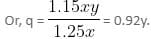As the new quantity that he can buy is 0.92y,
He gets 0.08y lesser than what he used to get earlier.
Or, a reduction of 8%.

Test Level 3: Percentages - Question 4

A company has raised seventy-five percent of the amount it needs for a new project by receiving an average amount of Rs. 600 from persons already associated. The already associated people represent 60% of the people from which the company will ask for the money. If the company needs exactly the same amount it needs for the project, then what is the average money it will get from the remaining people?

Detailed Solution for Test Level 3: Percentages - Question 4

Let x be the total number of people that will be asked for money.
Number of people already associated with the company = 0.6x
Number of remaining people = 0.4x
Total amount already collected = 0.6x × 600 = 360x
This amount represents 75% of total needed.
Company needs to raise 25% of total needed.
75% of total = 360x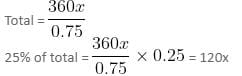So, the company needs to collect 120x from remaining 0.4x people to be solicited.
Average per person = 120x/0.4x = 300
So, average money collected from remaining people should be Rs. 300.

Test Level 3: Percentages - Question 5

In a group of p friends, x% have both credit cards and mobile phones. Out of p, y% of the males and z% of the females have both credit cards and mobile phones. Find the number of males in the group in terms of p, x, y and z.

Detailed Solution for Test Level 3: Percentages - Question 5

Total number of mobile phones and credit card holders = x% of p
Let the number of males be m.
So, the number of females = p - m
Thus, the number of mobile phones and credit cards held by males = y% of m
The number of mobile phones and credit cards held by females = z% of (p - m)
Thus, x% of p = y% of m + z% of (p - m)
Hence, m = p(x - z)/(y - z)
Thus, option (3) is the answer.

Test Level 3: Percentages - Question 6

Two candidates were contesting for the post of Rotary Club chairman. In the first round of voting, 550 members participated. In the second round, again 550 members participated. However, the number of members opposed to the first candidate increased by 150% and the combined votes of the first candidate (for both the rounds) exceeded that of the second by 400. How many members opposed the first candidate in the first round? No votes were invalid.

Detailed Solution for Test Level 3: Percentages - Question 6

Let in the 1st round, 'a' members voted for the 2nd candidate.
Thus, in the 1st round, members who voted for the 1st candidate = 550 - a
In the 2nd round, number of member who voted for the 2nd candidate = 2.5a
So, number of members who voted for the 1st candidate = 550 - 2.5a
Now, combined votes of both the rounds for the 1st candidate = 550 - a + 550 - 2.5a = 1100 - 3.5a
Combined votes of both the rounds for the 2nd candidate = a + 2.5a = 3.5a
Now, according to the question, 1100 - 3.5a - 3.5a = 400
Or, a = 100
Thus, 100 members opposed the 1st candidate in the 1st round.
Hence, answer option 4 is correct.

Test Level 3: Percentages - Question 7

In an exhibition, 60% of the collection is from the sales of cold drinks and rest is from the sales of water bottles. Had 30 more cold drinks been sold, their contribution would have been 80%. If the number of water bottles sold is  1/3rd of the number of cold drinks sold, find the total number of cold drinks sold.

Detailed Solution for Test Level 3: Percentages - Question 7

Let the number of cold drinks sold be '3x',
Then, number of water bottles sold = 1/3 of 3x = x
Let the price of each bottle of cold drink be 'c' and that of each water bottle be 'w'.
Total collection from the sales of cold drinks and water bottles = (3cx + wx)
60% of the collection is from sale of cold drinks (given).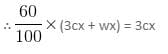This implies, 10cx = 6cx + 2wx
Therefore, 4cx = 2wx
This implies, w = 2c
Had 30 more cold drinks been sold, its contribution would have been 80%.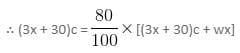This gives, 15cx + 150c = 12cx + 120c + 4wx
This implies, 15cx + 150c = 12cx + 120c + 4(2c)x
Hence, 5cx = 30c
This implies, x = 6
∴ Number of cold drinks sold = 3x = 3(6) = 18

Test Level 3: Percentages - Question 8

Padma purchased a box of chocolates. She ate 20% of them and gave 25% of the remaining chocolates to her friends. Again, she ate 20% of it and gave 25% of the remaining chocolates to her friends. Then, she gave 8 chocolates to a guest. Finally, she is left with 35% of the chocolates that she initially had. How many chocolates did Padma eat?

Detailed Solution for Test Level 3: Percentages - Question 8

Assume that the box contains x number of chocolates.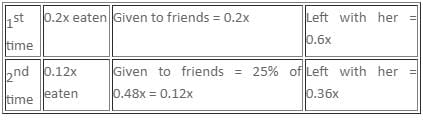Remaining = 0.36x
Given:
0.36x - 8 = 0.35x
x = 800
She ate 0.2x + 0.12x = 0.32x = 256 chocolates.

Test Level 3: Percentages - Question 9

In a container having milk, 20% milk is replaced by water and this process is repeated thrice. At the end of the third operation, the percentage of pure milk in the container is

Detailed Solution for Test Level 3: Percentages - Question 9

Let the total milk be 100ℓ.
After first operation, amount of pure milk = 100 - 20 = 80ℓ
After second operation, total solution = 100ℓ
(80ℓ milk + 20ℓ water)
Amount of milk taken out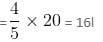Amount of pure milk left = 80 - 16 = 64ℓ
After third operation, total solution = 100ℓ(∵ of 64ℓ + 36ℓ)
Amount of milk taken out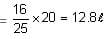Amount of remaining pure milk = 64 - 12.8 = 51.2ℓ

Test Level 3: Percentages - Question 10

A motorist uses 24% of his fuel in covering the first 20% of his total journey (in city driving conditions). He has to cover another 25% of his total journey in city driving conditions. What should be the minimum percentage increase in fuel efficiency for non-city driving over that in city driving, so that he is just able to cover his entire journey without having to refuel?

Detailed Solution for Test Level 3: Percentages - Question 10

He uses 24% fuel in covering 20% of the journey.
For next 25% of journey, he will consume 30% fuel.
So, for 45% of the journey in city driving conditions, 54% of the fuel is consumed.
Fuel efficiency = 45/54 = 5/6
Hence, for the remaining 55% journey, 46% fuel is left.
After increment, fuel efficiency = 55/46

## Level-wise Tests for CAT

5 docs|272 tests
 Use Code STAYHOME200 and get INR 200 additional OFF Use Coupon Code
Information about Test Level 3: Percentages Page
In this test you can find the Exam questions for Test Level 3: Percentages solved & explained in the simplest way possible. Besides giving Questions and answers for Test Level 3: Percentages, EduRev gives you an ample number of Online tests for practice

## Level-wise Tests for CAT

5 docs|272 tests

### How to Prepare for CAT

Read our guide to prepare for CAT which is created by Toppers & the best Teachers